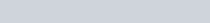ChemTalk

# Reaction Rates & How to Determine Rate Law## Core Concepts

Some reactions will go fast, and some will go slow – the speed of the reaction is it’s reaction rate, which is dictated by a rate law. In this article, we will learn about reaction rates, rate laws, the rate constant, and the reaction order.

The rate of a chemical reaction is determined—and altered—by many factors, including the nature (of reactivity) of reactants, surface area, temperature, concentration, and catalysts. For each unique chemical reaction, rate laws can be written at a rate law equation to show how the concentrations of reactants affect the rate of the reaction. It is important to note that you can only determine rate law experimentally!

## What is a Rate Law? The Rate Law Equation

The reaction rate can depend on how concentrated our reactants are. A chemical reaction’s rate law is an equation that describes the relationship between the concentrations of reactants in the reaction and the reaction rate. In the standard form, the rate law equation is written as: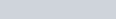•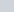is reaction rate, expressed in concentration/unit of time (usually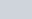= molarity/second)
•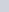is the specific rate constant
•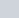and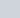are molar concentrations of reactants, expressed in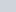(moles of solute/liters of solution)
•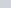and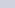are orders of the reaction

Let’s break down each of these components.

### Reaction Rate

As mentioned earlier, the rate of a reaction () is affected by many factors. This is why each chemical reaction has a unique rate law—each reaction has a different set of reactants, as well as different experimental conditions that affect the reaction rate.

Reaction rates are defined as the concentration of product that forms as the reaction progresses over time, so they are usually expressed in molarity/time in seconds ().

### Specific Rate Constant

Every reaction has its own constant in its rate equation. The specific rate constant () is a proportionality constant that is unique to each experimental reaction. This means that its value depends on other factors in the experiment that alter the reaction rate, such as temperature. Even with the same compounds used in a reaction,may change when other rate-altering factors change.

Furthermore, the units of the specific rate constant are dependent on the orders of the reaction. This will be discussed more in detail later.

### Molar Concentrations of Reactants

The rate law uses the molar concentrations of reactants to determine the reaction rate. Typically, increased concentrations of reactants increases the speed of the reaction, because there are more molecules colliding and reacting with each other.

The notation “” is read as “the molar concentration of Reactant A.”

The concentrations of reactants have units of molarity (), or moles of solute/liters of solution.

### Orders of Reactants & of the Reaction

The order of a reactant (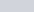) is the power to which the concentration of the reactant is raised to in the rate law equation. The order shows, mathematically, how the concentration of a reactant affects the rate law.

Let’s start with the most simple version of a rate law equation,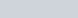When the order is 1, or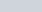, this means that the relationship between the concentration of Reactant A and the rate of the reaction is directly proportional. Whenincreases,will increase proportionally. Ifdoubles,doubles as well.

When the order is 2, or, this means that the rate of the reaction is directly proportional to the square of the concentration of Reactant A. Whenincreases,will increase, but not proportionally. For example, ifdoubles,will quadruple (because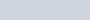.

When the order is 0, or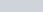, this means that the rate of reaction is not affected by any change in concentration of the reactant. This does not mean that the reactant is not needed; the reactant is still needed in the reaction, but the amount of reactant does not affect the rate of the reaction.

The total order of the reaction is the sum of all the orders of the reactants,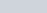.

## How to Determine the Rate Law

There are 2 main questions you’ll see when asked to determine the rate law. The first type asks you to find the rate law from elementary steps. The second type asks you to find the rate law from a table listing different experiments with different reactant concentrations and reaction rates.

Sometimes, you’ll have to find a rate law for a reaction with an intermediate. For that, you’ll need to find the rate determining step.

### From Elementary Steps

In many reactions, the chemical equation oversimplifies the reaction process. Usually, there are many intermediate reactions, or elementary steps, that occur to get from the reactants to the products.

For example, the equation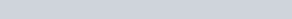is a series of 2 elementary steps:

1.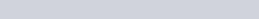2.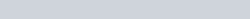When you add the steps together, you would get: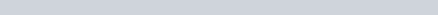.

The NO3 and NO2 cancel out on both sides of the equation, so you’re left with the original equation.

In these problems, you will usually be given the elementary steps and the rates of each of the steps. For example in the equation provided above, step 1 is the slow step, and step 2 is faster. The slower step is used as the rate-determining step—because the rate of reaction can only go as fast as the slowest step. You would use the rate-determining step to write the rate law by using its reactants.

(1)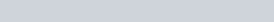The rate law does not include CO (the second reactant in the original chemical equation). This is because CO is not used in the slower, rate-determining step, so it does not affect the reaction rate.

### From a Table

To determine the rate law from a table, you must mathematically calculate how differences in molar concentrations of reactants affect the reaction rate to figure out the order of each reactant. Then, plug in values of the reaction rate and reactant concentrations to find the specific rate constant. Finally, rewrite the rate law by plugging in the specific rate constant and the orders for the reactants.

A table given will list the different tests of a reaction. Each different test will have different concentrations of reactants, and as a result, and the reaction rates for that test will be different. Here’s an example of a data table for the experiment: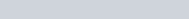In the experiment, hydrogen iodide HI is the reactant, and H2 and I2 are the products. From the table, you can tell that 3 experiments of the same reaction were run, with varying concentrations of HI. In each experiment, there reaction rate was different, as a result of the different concentrations of HI.

#### Determine Rate Law Using the Table

##### Finding the Order of Reactants

Going from experiment 1 to 2, you can see the concentration of HI was doubled (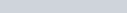). As a result (between those same experiments), the rate of reaction quadrupled (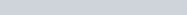). From this, you know the order of HI should be 2. The reason for this is that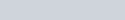, so. In other words, 22 = 4.

You can check this result using experiments 1 and 3 as well. Between these tests, the concentration of HI was tripled (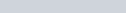). As a result, the rate of reaction was multiplied by a factor of 9 (). Since 32 = 9, you know that the order of HI is 2.

Mathematically, you can use the same process to find reactant orders by plugging values into the following equation: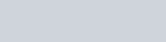With this equation, you are essentially using ratios of the rate law equation (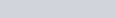) to find the orders of reactants. Plugging in values from the table above, you get: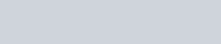Which simplifies to: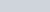, so. As expected, this is the same as the order you calculated earlier.

##### Finding the Specific Rate Constant

Now that you know the order of reactant HI, you can start to write the rate law. First, plug in the order into the rate law equation.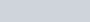Now you must find, the specific rate constant. Remember thatis unique to this experiment and this reaction. By plugging in the values of any of the experiments into the equation, you can find. If we plug in the values from experiment 1, we get: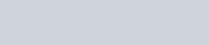So, the final rate law for this experiment is: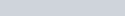##### Units for the Specific Rate Constant

As mentioned earlier, the units for the specific rate constant depend on the order of the reaction. Keep in mind:

• The unit of reaction rate is• The order of the reactant changes the units on the right side of the equation

For the example above,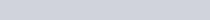, expanding the right side of the equation gives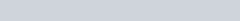. To isolate, you can divide both sides of the equation by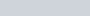to get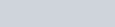. The units ofbecome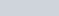.

However, in another (separate, unrelated) example, if the rate law were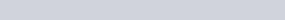, the units forwould be different. In this case, expanding the right side of the equation gives the units of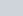on the right side. Isolating, the units ofwould be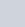, or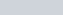.

As you can see, the order of each reactant affects the units of the specific rate constant.

## Example Questions for Determining Rate Law

### From Elementary Steps

Write the rate law for the following reaction given the reaction mechanism elementary steps: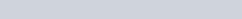1.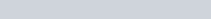2.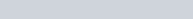Explanation: Since step 1 is the slower step, it is the rate-determining step for this reaction. Write the rate law by plugging in the reactants into the rate law equation.

Answer: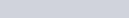### From a Table

Explanation:

To start, write the rate law for the equation:#### Finding the Orders of Reactants

Let’s start by finding the order of Reactant A. As you can see in the table, between experiments 1 and 2, the concentration of B changed, but the concentration of A did not—this would not be useful in finding the order of A. However, between experiments 1 and 3, the concentration of A changed, while B did not—this is perfect for finding the order of A because A is the only thing that changed, and therefore is the only variable that could have affected the reaction rate.Plugging in the values from the table, you get: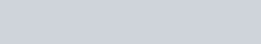As you can see, on the right side of the equation,and thevalues cancel out, isolating. Simplifying the equation, we get:, so. The order of A is 2.

Now, let’s find the order of Reactant B. As mentioned earlier, between experiments 1 and 2, the variable ofis isolated because it is the only variable being changed.Again, theandvalues cancel out. Simplifying the equation, we get: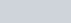, so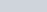. the order of B is 1.

*As a side note, you could also do this by comparing values on the table, without using the equation. Between experiments 1 and 2, as B was halved, the reaction rate was also halved. Thus, you know that the concentration of B had a directly proportional effect on the reaction rate, and the order of B was 1.

#### Finding the Specific Rate Constant

Now that you know the order of both reactants, plug them into the equation.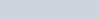Finally, find the value of k by plugging in the values from any of the experiments. If we choose to use experiment 1, we get: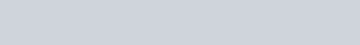#### Determine Rate Law Equation

So, the final rate law for this experiment is: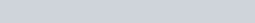## Rate Law Practice Problems

Problem 1

In an overall second-order reaction involving two reactants (Reactant X and Reactant Y), you observe the reaction rate double when you double the concentration of X. In another experiment, you halve the concentration of Y. How would you expect the reaction rate to change?

Problem 2

For the reaction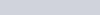, you observe the following data:

What is the rate law for this reaction?

## Rate Law Practice Problem Solutions

1: The rate would halve.

2: